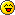Riddles :)

Poll

Do you like the riddles?

[ 1 ][100%]
[ 0 ][0%]XtremeX17
ModeratorNumber of posts : 135
Age : 23
Location : Dont turn around...
Your mood :Coins :Registration date : 2009-01-12

STUFF
STUFF: asd
1. Once upon a time, and old lady went to sell her vast quantity of eggs at the local market.

Son, I can't count past 100 but I know that.

If you divide the number of eggs by 2 there will be one egg left.
If you divide the number of eggs by 3 there will be one egg left.
If you divide the number of eggs by 4 there will be one egg left.
If you divide the number of eggs by 5 there will be one egg left.
If you divide the number of eggs by 6 there will be one egg left.
If you divide the number of eggs by 7 there will be one egg left.
If you divide the number of eggs by 8 there will be one egg left.
If you divide the number of eggs by 9 there will be one egg left.
If you divide the number of eggs by 10 there will be one egg left.

Finally. If you divide the Number of eggs by 11 there will be NO EGGS left!

How many eggs did the old lady have?

Spoiler:
25,201 eggs. This puzzle has a few different methods for finding the solution, one of which is:

Find a number X into which all of the numbers from 2 to 10 divide evenly. You can do this by simply using 2*3*4*5*6*7*8*9*10, but you can find a smaller number by finding the prime factors, a subset of which can be used to form any number from 2 to 10. 2*2*2*3*3*5*7 will do. This comes out to be 2520, and is the lowest number into which all the numbers 2-10 divide evenly.

We can add 1 to this number to satisfy the first 9 constraints of the puzzle (the remainder of 2521/2, 2521/3 ... 2521/10 is one), but this does not satisfy the last constraint, divisibility by 11.

Fortunately, we can multiply X (=2520) by any integer and add 1 and we will still satisfy constraints 1-9. So what Y do we multiply X by so that (X*Y) + 1 is divisible by 11. 2520/11 has a remainder of 1. Thus two 2520s divided by eleven would have a remainder of 1+1 = 2, and so forth...so ten 2520s divided by 11 would have a remainder of 10. This number plus one would divide eleven evenly, as well as also satisfy the first 9 constraints - thus 25201 is the answer.

2. I want to travel 1,000 miles on an adult tricycle and I have two spare tyres. However, I want to use all five tyres equally and have an equal mileage on each of the five tyres by the end of the trip. Of course I can only use three tyres at a time, so I will have to change tyres during the trip. At the end of the trip how many miles will each tyre have been used?

Spoiler:
600 miles: a total of 1,000 miles have been travelled and at any one time, three tyres are travelling. Therefore 3,000 tyre miles have been travelled, which is shared equally by the 5 tyres. QED.

3. Below you will find ten 6 letter words, however, every other letter is missing. Can you determine the words?

_s_e_d
_p_a_g
_r_f_r
_y_r_d
_r_f_e
_a_b_n
_a_e_a
_o_d_g
_s_f_l
_i_s_e

Spoiler:
Ascend, sprang, prefer, hybrid, trifle, carbon, camera, hotdog, useful, tissue.

4. I went on a trip last week. The traffic was moderate and the journey took two and a half hours. On the return journey, the traffic was similar, but I made it back in 150 minutes. How come?

Spoiler:
The times are the same!

These riddles aren't made by me !WeThEgReEnPeOpLeNumber of posts : 262
Age : 23
Your mood :Coins :Registration date : 2009-01-09

STUFF
STUFF: asd
xD these are good i did not get any of themi like them!

_________________
Before you criticize someone, walk a mile in their shoes. That way you're a mile away, and you have their shoes too.XtremeX17
ModeratorNumber of posts : 135
Age : 23
Location : Dont turn around...
Your mood :Coins :Registration date : 2009-01-12

STUFF
STUFF: asd
lol, i only got the last one